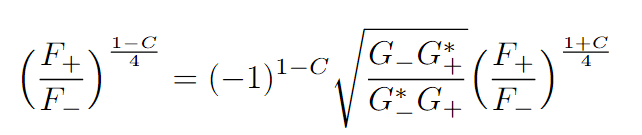# Simplifying a Tricky Equation

• I
• thatboi

#### thatboi

Hey all,
I am currently trying to solve the following equation for C:where C is a purely imaginary value, ##F_{+}##, ##F_{-}## and ##G_{+}## and ##G_{-}## are all complex valued constants (so ##G_{+}^{*}## just means complex conjugate of ##G_{+}##. I am not really sure where to start with isolating C, any advice would be greatly appreciated!

Take logarithms of both sides and see if you can solve that equation for C.

•Delta2 and malawi_glenn
Let ##F_+ /F_- = A## and ##\sqrt{ \dfrac{G_-G_+^*}{G_-^*G_+} } = B##

Your equation is ##A^{-C/2} = (-1)^{1-C}B ##

Always do simplifications and change of variables, to see what is going on.

Last edited:
•@thatboi , with the two hints given to you above, it is fairly easy to solve for C. Is that working out for you?

•malawi_glenn
phyzguy said:
@thatboi , with the two hints given to you above, it is fairly easy to solve for C. Is that working out for you?
Thanks for the hints I have already worked it out!

•malawi_glenn
Great!

May I ask where this equation came from?